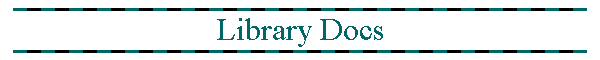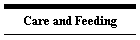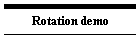# quaternions.gml File Reference

25/10/11 Important note: init_quaternion_random contained an error that caused it to output non-normalised results, and also probably skewed the distribution.

## Defines

#define  d3d_quaternion_set_projection_neg
#define  d3d_quaternion_set_projection
#define  d3d_quaternion_set_projection_neg_ext
#define  d3d_quaternion_set_projection_ext
#define  d3d_quaternion_light_direction_neg
#define  d3d_quaternion_light_direction
#define  d3d_set_rotation_quaternion
#define  quaternion_ball_roll_simple
#define  init_quaternion
#define  init_quaternion_random
#define  normalise_quaternion
#define  quaternion_fly_roll
#define  quaternion_fly_pitch
#define  quaternion_fly_yaw
#define  quaternion_rotate_vector
#define  sinc
#define  multiply_quaternion
#define  multiply_quaternion_left
#define  multiply_quaternion_right## Detailed Description

A collection of Gamemaker scripts for rotation using simplified quaternion algebra.

Quaternions are derived from complex numbers, and whereas a unit complex number can describe an orientation in a 2D space a quaternion can describe any orientation in a 3D space and provides a code-efficient means to transform orientations particularly in Gamemaker where the more usual matrix algebra would require a larger number of operations for the same result

Quaternions have many of the characteristics of rotation matrices and indeed the same results could be obtained from matrices. In my opinion quaternions are preferable in Gamemaker as the gamemaker language does not have vectorised math functions (matrix functions) or an effective way to feed a rotation matrix into Direct3D.

Quaternions are used routinely in 3D modeling as they provide an efficient way to store an orientation.## Define Documentation

Function to apply quaternion q0,q1,q2,q3 to the current drawing state using a rotation about an axis. The function uses d3d_transform_add_rotation_axis

note that although I do not have mathematical proof it appears as though any orientation may be expressed as a single rotation about an axis, and therefore that any combination of rotations about multiple axes may be combined into one rotation about new axis

 #define d3d_quaternion_light_direction

Arguments are ind,q0,q1,q2,q3,color

Applies a directional light according to a quaternion

Script treats x as the base direction.

 #define d3d_quaternion_light_direction_neg

Arguments are ind,q0,q1,q2,q3,color

Applies a directional light according to a quaternion

Script treats -x as the base direction.

 #define d3d_quaternion_set_projection

Set a camera orientation from a quaternion This script treats +x as the base direction, eg looking RIGHT on the map, to be consistant with the rest of Gamemaker

 #define d3d_quaternion_set_projection_ext

) d3d_quaternion_set_projection_ext(x,y,z,q0,q1,q2,q3,angle,aspect,znear ,zfar)

Set a camera orientation from a quaternion using the extended form This script treats +x as the base direction, eg looking RIGHT on the map, to be consistant with the rest of Gamemaker

Derived from d3d_quaternion_set_projection_neg();

 #define d3d_quaternion_set_projection_neg

Set a camera orientation from a quaternion This script treats -x as the base direction, eg looking LEFT on the map, which is inconsistant with the rest of Gamemaker but was convenient in d3d as on a textured sphere the middle of the texture faces left. This function will be replaced, for consistancy with 2D games where zero degrees means looking right.

 #define d3d_quaternion_set_projection_neg_ext

) d3d_quaternion_set_projection_neg_ext(x,y,z,q0,q1,q2,q3,angle,aspect,znear ,zfar)

Set a camera orientation from a quaternion using the extended form This script treats -x as the base direction, eg looking LEFT on the map, which is inconsistant with the rest of Gamemaker but was convenient in d3d as on a textured sphere the middle of the texture faces left. This function will be replaced, for consistancy with 2D games where zero degrees means looking right.

Derived from d3d_quaternion_set_projection_neg();

 #define d3d_set_rotation_quaternion

Function to apply quaternion q0,q1,q2,q3 to the current drawing state using a rotation about an axis. The function uses d3d_transform_set_rotation_axis

 #define init_quaternion

Sets the variables q0,q1,q2,q3 to the basic unit quaternion (1,0,0,0);

 #define init_quaternion_random

Sets the variables q0,q1,q2,q3 to a random orientation quaternion. This is done by generating random angles and converting the result to a quaternion note the special distribution of "theta" needed to prevent bias towards certain orientations

Picture a globe, phi is longitude, theta is latitude and psi is which way you are facing. Phi and Psi can be simply random but if theta is a simple random number then if you are near the poles the lines of longitude are closer together. This skews things

Starting from the equator on a radius 1 globe the area of the northen hemisphere is 2*pi and the length of a line of latitude is 2*pi*cos(theta). The area between the equator is 2*pi*sin(theta) (basic integral, only works in radians otherwise it gets ugly) and from this I infer that sin(theta) should have a flat distribution and so if theta=arcsin(random) then sin(theta)=sin(arcsin(random)) so sin(theta)=random (provided random lies in the range -1 to +1.

25/10/11 Important note: The version of this function previously available has a typo:

multiply_quaternion_right(cos(psi/2),0,0,sin(psi/2)); //<==typo, psi NOT phi

 #define multiply_quaternion

multiply two quaternions r and s arguments are real,i,j,k,real2,i2,j2,k2 result is returned in variables q0,q1,q2,q3

note that the order of the two quaternions is very important. multiply_quaternion(s,r) is not the same as multiply_quaternion(r,s)

 #define multiply_quaternion_left

multiply two quaternions l (from arguments) and q (from object) arguments are real,i,j,k parts of quaternion

note that the order of the two quaternions is very important. multiply_quaternion_left will apply a rotation in the room's reference frame not the object's frame.

 #define multiply_quaternion_right

multiply two quaternions q (from object) and r (from arguments) arguments are real,i,j,k parts of quaternion result is returned in variables q0,q1,q2,q3

note that the order of the two quaternions is very important. multiply_quaternion_right will apply a rotation in the object's reference frame

 #define normalise_quaternion

Normalises (q0,q1,q2,q3) Ensures that math errors have not shifted the absolute value away from 1. Note that this function uses slow math functions but the optimised math form uses more code and so would run slower in Gamemaker.

 #define quaternion_ball_roll_simple

This function implements a simple rolling motion by converting the object's hspeed,vspeed into a rotation and applying that to the object's orientation (q0,q1,q2,q3).

 #define quaternion_fly_pitch

pitch manouver script for aircraft-like movement

causes an object to pitch by an amount angle

see quaternion_fly_roll() for details

 #define quaternion_fly_roll

roll manouver script for aircraft-like movement

causes an object to roll by an amount angle

using multiply_quaternion_right() causes the rotation to be carried out in the object's reference frame instead of the room's frame

 #define quaternion_fly_yaw

yaw manouver script for aircraft-like movement

causes an object to yaw by an amount angle

see quaternion_fly_roll() for details

 #define quaternion_rotate_vector

script to rotate a vector by an object's quaternion

arguments are the x,y,z components of the vector the result is put into variables vx,vy,vz

this can be called with (1,0,0) to obtain a vector pointing the way the object is facing. Use (-1,0,0) if you are using the _neg functions

 #define sinc

This function is defined as sin(x)/x It is used by the quaternion_ball_roll_simple() function as part of the formula for converting a vector into a rotation axis because it is valid for x=0 and so it won't cause divide by zero errors

note that for very small x sinc(x) is close to 1-sqr(x)/6 and when x is effectively zero sinc(x) is defined as being 1